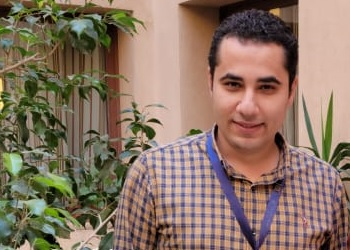Position
Visiting Assistant Professor
Department
Department of Mathematics and Actuarial Science

# Profile

Brief Biography

Eslam Badr earned his PhD from Universitat Autonoma de Barcelona (UAB) in Spain before joining the AUC. His area of expertise is within algebraic geometry and arithmetic. More specifically, his research mainly deals with explicit geometric and arithmetic properties of smooth plane curves/hypersurfaces and their moduli spaces. Topics including but not limited to: automorphism groups, twisting theory, fields of definition versus the field of moduli, quadratic points and geometric progression sequences.

Badr is interested in teaching several mathematics courses including, Algebra and Mathematical Logic, Differential and Integral Calculus, Linear and Abstract Algebra, Ordinary Differential Equations, Galois Theory, Number Theory and, Arithmetic geometry.

Research Interest
• Automorphism groups
• Twisting theory
• Fields of definition versus the field of moduli
• Quadratic points and geometric progression sequences
• Weierstrass points